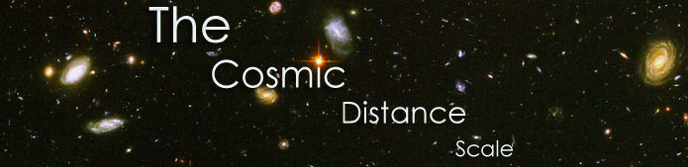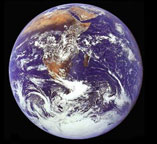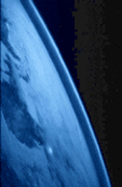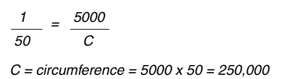# National Aeronautics and Space Administration

## Goddard Space Flight Center# The EarthImage Credit: NASA, Apollo 17, NSSDC

The crew of Apollo 17 took this photograph of Earth in December 1972 while their spacecraft was traveling between the Earth and the Moon. The orange-red deserts of Africa and Saudi Arabia stand in stark contrast to the deep blue of the oceans and the white of both clouds and snow-covered Antarctica.

### Distance Information

The diameter of the Earth at the equator is 12,756 kilometers (km).

### How Do We Calculate Distances of This Magnitude?Image Credit: Clementine, Naval Research Laboratory. It is copyright free.

In 200 B.C., the size of the Earth was actually calculated to within 1% accuracy! Eratosthenes used Aristotle's idea that, if the Earth was round, distant stars in the night sky would appear at different positions to observers at different latitudes. Eratosthenes knew that on the first day of summer, the Sun passed directly overhead at Syene, Egypt. At midday of the same day, he measured the angular displacement of the Sun from overhead at the city of Alexandria - 5000 stadia away from Syene. He found that the angular displacement was 7.2 degrees - there are 360 degrees in a circle, making 7.2 degrees equivalent to 1/50 of a circle. Geometry tells us that the ratio of 1/50 is the same as the ratio of the distance between Syene and Alexandria to the total circumference of the Earth. Thus the circumference can be estimated by multiplying the distance between the two cities, 5000 stadia, by 50, equaling 250,000 stadia.How do we convert to kilometers? Well, we believe that the unit of the "stadium" was about 0.15 km. This means that Eratosthenes estimated the circumference of the Earth to be about 40,000 km. He also knew that the circumference of a circle was equal to 2 times π (3.1415...) times the radius of the circle. (C = 2πr) With this information, Eratosthenes inferred that the Earth's radius was 6366 km. Both of these values are very close to the accepted modern values for the Earth's circumference and radius, 40,070 km and 6378 km respectively, which have since been measured by orbiting spacecraft.

The diameter of a circle is twice the radius, giving us a diameter for Earth of 12,756 km.

Note: The Earth is almost, but not quite, a perfect sphere. Its equatorial radius is 6378 km, but its polar radius is 6357 km - in other words, the Earth is slightly flattened. Eratosthenes was measuring the polar radius, and his value (using the 0.15 km/stadium conversion) lies between the polar and equatorial values.

### Why Are These Distances Important To Astronomers?

Duringthe 18th and 19th centuries, astronomers used the diameter of the Earth as the basic yardstick in determining the size of the solar system. Today's astronomers usually do not need to know the size of the Earth for their daily research activities. Nevertheless, the diameter of the Earth still is the first step for us, the residents of this planet, in our attempt to understand the cosmic distance scale.

### Travel Time

The Space Shuttle orbiter, with a velocity of 27,880 km per hour (17,322 miles per hour) takes approximately 90 minutes to orbit, or travel just outside the circumference of the Earth. At an altitude of 322 km (200 miles), an orbiter covers approximately 41,300 km (26,000 miles) in one orbit.

Back